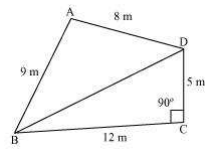# A park, in the shape of a quadrilateral ABCD, has C = 90°, AB = 9 m, BC = 12 m, CD = 5 m and AD = 8 m. How much area does it occupy?

Given

A quadrilateral ABCD where ∠C = 90º, AB = 9 m, BC = 12 m, CD = 5 m and AD = 8 m

Join BDFind out

We have to determine the area of the quadrilateral

Formula

By using Heron’s formula, area of triangle= $$\sqrt{s(s-a)(s-b)(s-c)}$$

Solution

On applying Pythagoras theorem to triangle BCD we get

BD2 = BC+CD2

⇒ BD2 = 122+52

⇒ BD2 = 169

⇒ BD = 13 m

Now, the area of ΔBCD = (½ ×12×5) = 30 m2

The semi perimeter of ΔABD

(s) = (perimeter/2)

= (8+9+13)/2 m

= 30/2 m = 15 m

Using Heron’s formula,

Area of ΔABD= $$\sqrt{15(15-13)(15-9)(15-8)}$$

= 6√35 m= 35.5 m2 (approx)

∴ The area of quadrilateral ABCD = Area of ΔBCD+Area of ΔABD

= 30 m2+35.5m2 = 65.5 m2

Hence, the area of quadrilateral= 65.5 m2